# Frank solutions for Class 9 Maths ICSE chapter 19 - Quadrilaterals [Latest edition]

#### Chapters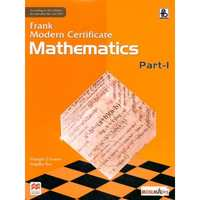Exercise 19.1Exercise 19.2
Exercise 19.1

### Frank solutions for Class 9 Maths ICSE Chapter 19 Quadrilaterals Exercise 19.1

Exercise 19.1 | Q 1.1

In the following figures, find the remaining angles of the  parallelogram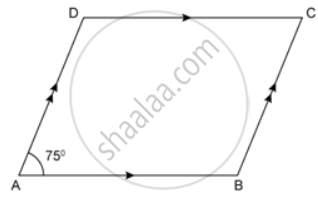Exercise 19.1 | Q 1.2

In the following figures, find the remaining angles of the  parallelogram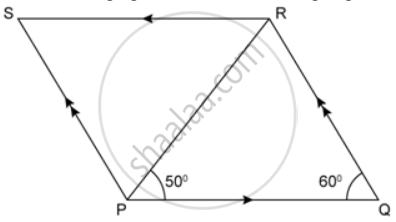Exercise 19.1 | Q 1.3

In the following figures, find the remaining angles of the  parallelogram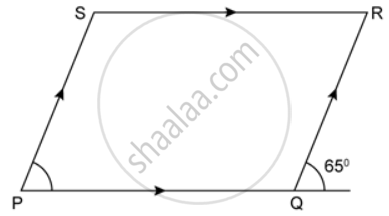Exercise 19.1 | Q 1.4

In the following figures, find the remaining angles of the  parallelogram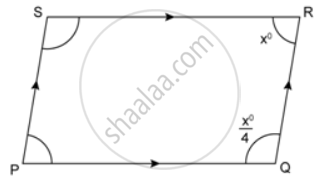Exercise 19.1 | Q 1.5

In the following figures, find the remaining angles of the  parallelogram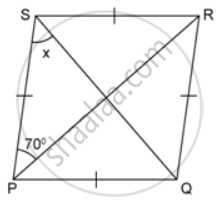Exercise 19.1 | Q 2

In a parallelogram ABCD ∠C = 98°. Find ∠A and ∠B.

Exercise 19.1 | Q 3

The consecutive angles of a parallelogram are in the ratio 3:6. Calculate the measures of all the angles of the parallelogram.

Exercise 19.1 | Q 4

Find the measures of all the angles of the parallelogram shown in the figure: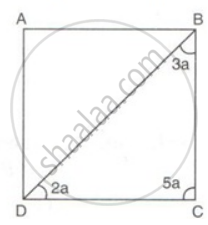Exercise 19.1 | Q 5

In the given figure, ABCD is a parallelogram, find the values of x and y.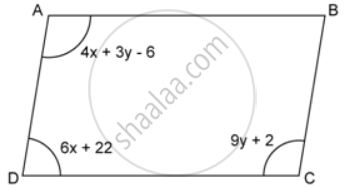Exercise 19.1 | Q 6

The angles of a triangle formed by 2 adjacent sides and a diagonal of a parallelogram are in the ratio 1 : 5 : 3. Calculate the measures of all the angles of the parallelogram.

Exercise 19.1 | Q 7

PQR is a triangle formed by the adjacent sides PQ and QR and diagonal PR of a parallelogram PQRS. If in ΔPQR, ∠P : ∠Q : ∠R = 3 : 8 : 4, Calculate the measures of all the angles of parallelogram PQRS.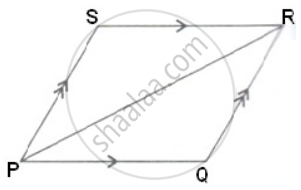Exercise 19.1 | Q 8.1

PQRS is a parallelogram. T is the mid-point of PQ and ST bisects ∠PSR.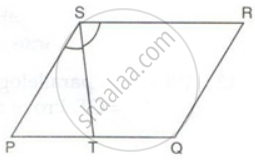Prove that: QR = QT

Exercise 19.1 | Q 8.2

PQRS is a parallelogram. T is the mid-point of PQ and ST bisects ∠PSR.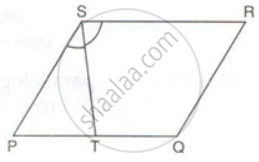Prove that: RT bisects angle R

Exercise 19.1 | Q 8.3

PQRS is a parallelogram. T is the mid-point of PQ and ST bisects ∠PSR.Prove that: ∠RTS = 90°

Exercise 19.1 | Q 9.1

ABCD is a parallelogram. The bisector of ∠BAD meets DC at P, and AD is half of AB.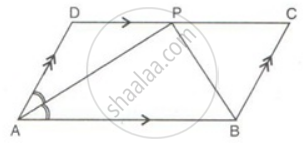Prove that: BP bisects ∠ABC.

Exercise 19.1 | Q 9.2

ABCD is a parallelogram. The bisector of ∠BAD meets DC at P, and AD is half of AB.Prove that: ∠APB is a right angle.

Exercise 19.1 | Q 10

ABCD is a parallelogram. P and Q are mid-points of AB and CD. Prove that APCQ is also a parallelogram.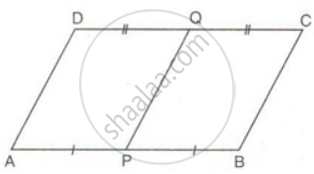Exercise 19.1 | Q 11

SN and QM are perpendiculars to the diagonal PR of parallelogram PQRS.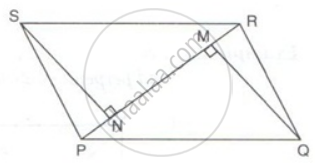Prove that:
(i) ΔSNR ≅ ΔQMP
(ii) SN = QM

Exercise 19.1 | Q 12

Points M and N are taken on the diagonal AC of a parallelogram ABCD such that AM = CN. Prove that BMDN is a parallelogram.

Exercise 19.1 | Q 13

In the given figure, MP is the bisector of ∠P and RN is the bisector of ∠R of parallelogram PQRS. Prove that PMRN is a parallelogram.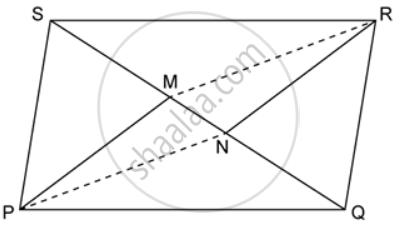Exercise 19.1 | Q 14

ABCD is a parallelogram. P and T are points on AB and DC respectively and AP = CT. Prove that PT and BD bisect each other.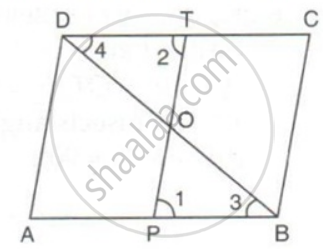Exercise 19.1 | Q 15

PQRS is a parallelogram. PQ is produced to T so that PQ = QT. Prove that PQ = QT. Prove that ST bisects QR.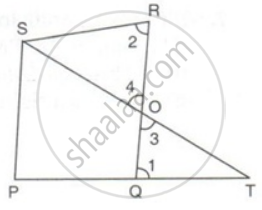Exercise 19.2

### Frank solutions for Class 9 Maths ICSE Chapter 19 Quadrilaterals Exercise 19.2

Exercise 19.2 | Q 1

PQRS is a square whose diagonals PR and QS intersect at O.M is a point on QR such that OQ = MQ. Find the measures of ∠MOR and ∠QSR.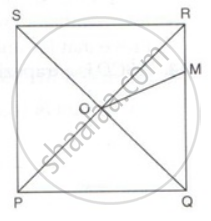Exercise 19.2 | Q 2

ABCD is a rectangle with ∠ADB = 55°, calculate ∠ABD.

Exercise 19.2 | Q 3

Prove that if the diagonals of a parallelogram are equal then it is a rectangle.

Exercise 19.2 | Q 4

Prove that the quadrilateral formed by joining the mid-points of a square is also a square.

Exercise 19.2 | Q 5

The diagonals PR and QS of a quadrilateral PQRS are perpendicular to each other. A, B, C and D are mid-point of PQ, QR, RS and SP respectively. Prove that ABCD is a rectangle.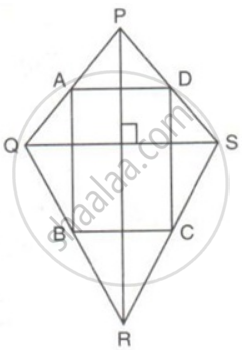Exercise 19.2 | Q 6

ABCD is a quadrilateral P, Q, R and S are the mid-points of AB, BC, CD and AD. Prove that PQRS is a parallelogram.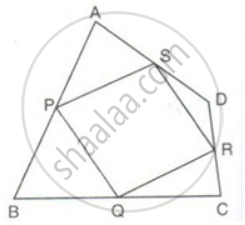Exercise 19.2 | Q 7

Prove that the quadrilateral formed by joining the mid-points of consecutive sides of a rectangle is a rhombus.

Exercise 19.2 | Q 8

Prove that the quadrilateral formed by joining the mid-points of consecutive sides of a rhombus is a rectangle.

Exercise 19.2 | Q 9

PQRS is a parallelogram. T is the mid-point of RS and M is a point on the diagonal PR such that MR = (1)/(4)"PR". TM is joined and extended to cut QR at N. Prove that QN = RN.

Exercise 19.2 | Q 10

P is a point on side KN of a parallelogram KLMN such that KP : PN is 1 : 2. Q is a point on side LM such that LQ : MQ is 2 : 1. Prove that KQMP is a parallelogram.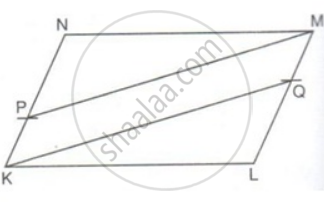Exercise 19.2 | Q 11

PQRS is a parallelogram. M and N are the mid-points of the adjacent sides QR and RS. O is the mid-point of the diagonal PR. Prove that MONR is a rectangle and MN is half of PR.

Exercise 19.2 | Q 12

Prove that the line segment joining the mid-points of the diagonals of a trapezium is parallel to each of the parallel sides, and is equal to half the difference of these sides.

Exercise 19.2 | Q 13.1

In a parallelogram PQRS, M and N are the midpoints of the opposite sides PQ and RS respectively. Prove that
RN and RM trisect QS.

Exercise 19.2 | Q 13.2

In a parallelogram PQRS, M and N are the midpoints of the opposite sides PQ and RS respectively. Prove that
PMRN is a parallelogram.

Exercise 19.2 | Q 13.3

In a parallelogram PQRS, M and N are the midpoints of the opposite sides PQ and RS respectively. Prove that
MN bisects QS.

Exercise 19.2 | Q 14

ABCD is a trapezium in which side AB is parallel to side DC. P is the mid-point of side AD. IF Q is a point on the Side BC such that the segment PQ is parallel to DC, prove that PQ = (1)/(2)("AB" + "DC").

Exercise 19.2 | Q 15.1

In the given figure, PQRS is a parallelogram in which PA = AB = Prove that: SA ‖ QB and SA = QB.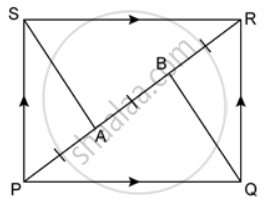Exercise 19.2 | Q 15.2

In the given figure, PQRS is a parallelogram in which PA = AB = Prove that: SAQB is a parallelogram.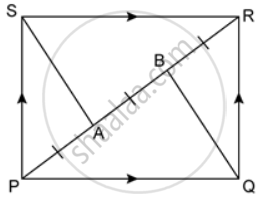Exercise 19.2 | Q 16

In the given figure, PQRS is a trapezium in which PQ ‖ SR and PS = QR. Prove that: ∠PSR = ∠QRS and ∠SPQ = ∠RQP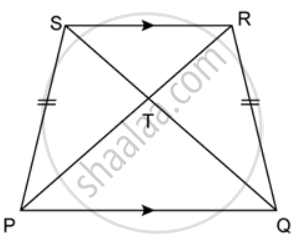Exercise 19.2 | Q 17.1

In a parallelogram ABCD, E is the midpoint of AB and DE bisects angle D. Prove that: BC = BE.

Exercise 19.2 | Q 17.2

In a parallelogram ABCD, E is the midpoint of AB and DE bisects angle D. Prove that:CE is the bisector of angle C and angle DEC is a right angle

Exercise 19.2 | Q 18

Prove that if the diagonals of a quadrilateral bisect each other at right angles then it is a rhombus.

Exercise 19.2 | Q 19

Prove that the diagonals of a kite intersect each other at right angles.

Exercise 19.2 | Q 20

Prove that the diagonals of a square are equal and perpendicular to each other.

Exercise 19.1Exercise 19.2## Frank solutions for Class 9 Maths ICSE chapter 19 - Quadrilaterals

Frank solutions for Class 9 Maths ICSE chapter 19 (Quadrilaterals) include all questions with solution and detail explanation. This will clear students doubts about any question and improve application skills while preparing for board exams. The detailed, step-by-step solutions will help you understand the concepts better and clear your confusions, if any. Shaalaa.com has the CISCE Class 9 Maths ICSE solutions in a manner that help students grasp basic concepts better and faster.

Further, we at Shaalaa.com provide such solutions so that students can prepare for written exams. Frank textbook solutions can be a core help for self-study and acts as a perfect self-help guidance for students.

Concepts covered in Class 9 Maths ICSE chapter 19 Quadrilaterals are Introduction of Rectilinear Figures, Names of Polygons, Concept of Quadrilaterals - Sides, Adjacent Sides, Opposite Sides, Angle, Adjacent Angles and Opposite Angles, Diagonal Properties of Different Kinds of Parallelograms, Property: The Diagonals of a Rectangle Are of Equal Length., Property: The diagonals of a square are perpendicular bisectors of each other., Types of Quadrilaterals, Property: The Opposite Sides of a Parallelogram Are of Equal Length., Property: The Opposite Angles of a Parallelogram Are of Equal Measure., Property: The diagonals of a rhombus are perpendicular bisectors of one another..

Using Frank Class 9 solutions Quadrilaterals exercise by students are an easy way to prepare for the exams, as they involve solutions arranged chapter-wise also page wise. The questions involved in Frank Solutions are important questions that can be asked in the final exam. Maximum students of CISCE Class 9 prefer Frank Textbook Solutions to score more in exam.

Get the free view of chapter 19 Quadrilaterals Class 9 extra questions for Class 9 Maths ICSE and can use Shaalaa.com to keep it handy for your exam preparation